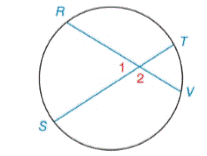Chapter 6.2, Problem 15EElementary Geometry For College St...

7th Edition
Alexander + 2 others
ISBN: 9781337614085

Solutions

Chapter
SectionElementary Geometry For College St...

7th Edition
Alexander + 2 others
ISBN: 9781337614085
Textbook Problem

Given: m ∠ 2 = 124 ° m T V ⌢ = x + 1 m S R ⌢ = 3 ( x + 1 ) Find: m T V ⌢Exercises 14, 15

To determine

To find:

mTV when m2=124°,mVT=x+1, mSR=3(x+1)

Explanation

m2=124°m1+m2=180°m1+124°=180°m1=180°124°m1=56°

Theorem:

The measure of an angle formed by two chords that intersect within a circle is one-half the sum of the measures of the arcs intercepted by the angle and its vertical angle.

mTV when m1=56°,mVT=x+1, mSR=3(x+1)

Still sussing out bartleby?

Check out a sample textbook solution.

See a sample solution

The Solution to Your Study Problems

Bartleby provides explanations to thousands of textbook problems written by our experts, many with advanced degrees!

Get Started

In Exercises 7-12, solve for y in terms of x. 5y6x21=0

Calculus: An Applied Approach (MindTap Course List)

In Problems 13-26, graph the solution of each system of inequalities. 24.

Mathematical Applications for the Management, Life, and Social Sciences

Simplify the expressions in Exercises 97106. x1/2y2x1/2y

Finite Mathematics and Applied Calculus (MindTap Course List)

Evaluate the definite integral. 0axx2+a2dx(a0)

Single Variable Calculus: Early Transcendentals, Volume I

Simplify: 2025

Elementary Technical Mathematics

Use a Table of Integrals to evaluate .

Study Guide for Stewart's Single Variable Calculus: Early Transcendentals, 8th